﻿ 基于改进PSO的视频传感器网络覆盖增强算法

# 基于改进PSO的视频传感器网络覆盖增强算法An Effective Coverage Enhancement Method for Video Sensor Network Based on Improved PSO

Abstract: Coverage rate can reflect the sensing quality of sensor networks. It is the foundation and precondition for other related researches. The unchangeable parameters of traditional PSO algorithm may lead to slow convergent speed or being unable to converge to the optimal solution. In this paper, an improved PSO is proposed and applied to the coverage enhancement of video sensor network. By improving the inertia weight and learning factors, the algorithm has larger inertia at starting period. The nodes search in the local region with bigger steps and improve the convergent speed. At later stage, smaller inertia will lead to the algorithm searches near the optimal point with small steps. Then, the algorithm has high possibility to converge to the global optimal solution and improve the coverage rate. Experimental results show that the proposed method has high converging speed, and the coverage rate is improved effectively.

1. 引言

2. 基于PSO算法的有向传感器网络覆盖增强

2.1. 视频传感器网络模型

$CR=\frac{CA}{TA}$ (1)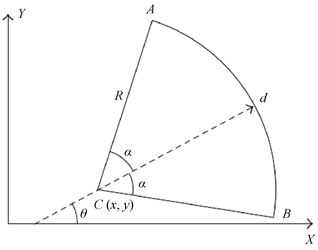Figure 1. Model of video sensor node

2.2. 基于PSO的覆盖增强方法

${v}_{i}\left(t+1\right)=w{v}_{i}\left(t\right)+{c}_{1}{r}_{1}\left({P}_{i}-{p}_{i}\left(t\right)\right)+{c}_{2}{r}_{2}\left({G}_{best}-{p}_{i}\left(t\right)\right)$ (2)

${x}_{i}\left(t+1\right)={x}_{i}\left(t\right)+{v}_{i}\left(t+1\right)$ (3)

3. 改进PSO算法及其应用

3.1. 改进的PSO算法

${c}_{1}={c}_{1\mathrm{max}}-t\ast \left({c}_{1\mathrm{max}}-{c}_{1\mathrm{min}}\right)/MaxIT$ (4)

${c}_{2}={c}_{2\mathrm{min}}+t\ast \left({c}_{2\mathrm{max}}-{c}_{2\mathrm{min}}\right)/MaxIT$ (5)

$w\left(t\right)={w}_{\mathrm{max}}-\frac{\left({w}_{\mathrm{max}}-{w}_{\mathrm{min}}\right)×t}{MaxIT}$ (6)

3.2. 本文视频传感器网络覆盖增强算法步骤

4. 实验及分析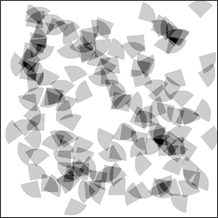(a)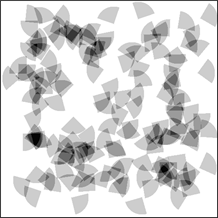(b)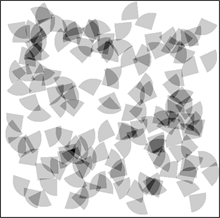(c)

Figure 2. Coverage results. (a) Original distribution; (b) Coverage result of ; (c) Result of proposed method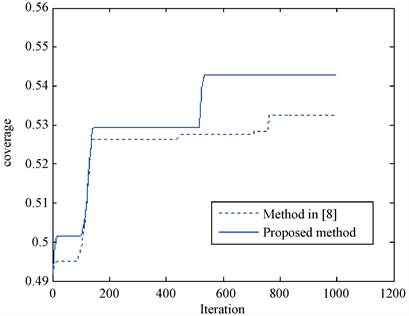Figure 3. Curves between coverage rate and iteration timeTable 1. Coverage rate and convergent time with different number of nodes

5. 结论

 蒋一波, 何成龙, 梅佳东, 汪念华. 基于不规则划分的K级区域覆盖增强算法[J]. 计算机科学, 2019, 46(5): 67-72.

 周挺. 全向传感器网络中基于虚拟力的三维覆盖增强算法[J]. 内蒙古师范大学学报(自然科学汉文版), 2017, 46(4): 566-570.

 Sung, T.-W. and Yang, C.-S. (2014) Voronoi-Based Coverage Improvement Approach for Wireless Directional Sensor Networks. Journal of Network and Computer Applications, 39, 202-213.
https://doi.org/10.1016/j.jnca.2013.07.003

 Kong, L.H., Zhao, M.C., Liu, X.Y. and Lu, J.L. (2014) Surface Coverage in Sensor Networks. IEEE Transaction on Parallel and Distributed Systems, 25, 234-243.
https://doi.org/10.1109/TPDS.2013.35

 Ma, H.D. and Liu, Y.H. (2005) On Coverage Problems of Directional Sensor Networks. International Conference on Mobile Ad2hoc and Sensor Networks, Wuhan, 13-15 December 2005, 721-731.
https://doi.org/10.1007/11599463_70

 郭绪坤, 范冰冰, 陈纯炼, 孙纲. 基于虚拟仿真势场的无线视频传感器网均衡协议[J]. 计算机应用研究, 2017, 34(4): 1195-1198+1212.

 谭励, 杨朝玉, 杨明华, 唐小江. 有向移动传感器网络三维空间目标自主覆盖算法[J]. 计算机工程, 2018, 44(5): 71-77.

 王昌征, 毛剑琳, 付丽霞, 郭宁, 曲蔚贤. 有向异构传感器网络覆盖优化算法[J]. 传感器与微系统, 2016, 35(11): 132-135.

 Xu, Y.C., Lei, B.J. and Hendriks, E.A. (2011) Camera Network Coverage Improving by Particle Swarm Optimization. Journal on Image and Video Processing, No. 3, 1-10.
https://doi.org/10.1155/2011/458283

 张聚伟, 王宇. 基于PSO的有向传感器网络覆盖增强策略及仿真[J]. 系统仿真学报, 2017, 29(1): 181-189.

 Dai, Y.S. and Niu, H. (2011) An Improved PSO Algorithm and its Application in Seismic Wavelet Extraction. International Journal of Intelligent Systems and Applications, 5, 34-40.
https://doi.org/10.5815/ijisa.2011.05.05

Top